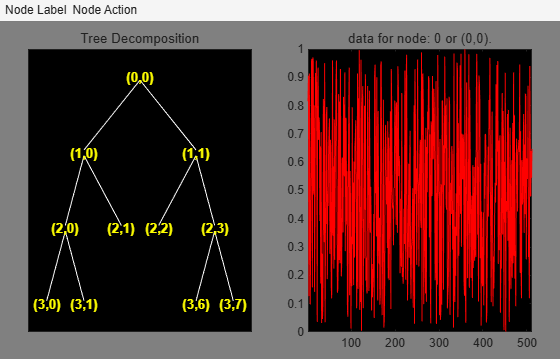## Syntax

``value = read(T,propname,propparam)``

## Description

example

````value = read(T,propname,propparam)` returns the value of the wavelet packet tree `T` property specified by `propname`. `propparam` is an optional parameter depending on the value of `propname`.You can specify one or more properties in any order. `propname-propparam` arguments must appear after other arguments. For example, ```[value1,value2,value3,value4] = read(T,propname1,propname2,propname3,propparam3,propname4,propparam4)```.```

## Examples

collapse all

Create a wavelet packet tree.

```x = rand(1,512); t = wpdec(x,3,"db3"); t = wpjoin(t,[4;5]);```

Display the tree.

`plot(t)`Obtain the size of the data at the nodes.

`sAll = read(t,"sizes")`
```sAll = 11×2 1 512 1 258 1 258 1 131 1 131 1 131 1 131 1 68 1 68 1 68 ⋮ ```
`sNod = read(t,"sizes",[0,4,5])`
```sNod = 3×2 1 512 1 131 1 131 ```

Obtain the entropy.

`eAll = read(t,"ent")`
```eAll = 11×1 116.3597 45.9147 39.1646 -30.2074 17.6607 20.8560 18.8364 -114.8913 11.4664 9.2578 ⋮ ```
`eNod = read(t,"ent",[0,4,5])`
```eNod = 3×1 116.3597 17.6607 20.8560 ```

Obtain the wavelet filters and wavelet coefficients.

```[loD,hiD,loR,hiR] = read(t,"wfilters"); [loD1,loR1,hiD1,hiR1] = read(t,"wfilters","l","wfilters","h"); [max(abs(loD-loD1)) max(abs(hiD-hiD1)) ... max(abs(loR-loR1)) max(abs(hiR-hiR1))]```
```ans = 1×4 0 0 0 0 ```
```dAll = read(t,"data"); dNod = read(t,"data",[4;5]); [ent,cfs4,cfs5] = read(t,"ent","cfs",4,"cfs",5); [max(abs(dNod{1}-cfs4)) max(abs(dNod{2}-cfs5))]```
```ans = 1×2 0 0 ```
```plot(cfs4) title("Node 4 Wavelet Coefficients")```## Input Arguments

collapse all

Wavelet packet tree, specified as a `WPTREE` object.

Wavelet packet tree property name, specified as one of the following:

Property Name (`propname`)

Property Parameter (`propparam`)

`"ent"`, `"ento"` or `"sizes"` (see `wptree`)

Without `propparam` or with `propparam` = Vector of node indices, `value` contains the entropy (or optimal entropy, or size) of the tree nodes in ascending node index order.

`"cfs"`

With `propparam` = One terminal node index. ```value = read(T,"cfs",NODE)``` is equivalent to `value = read(T,"data",NODE)` and returns the coefficients of the terminal node `NODE`.

`"entName"`,``` "entPar"```, `"wavName"` (see `wptree`) or `"allcfs"`

Without `propparam`. `value = read(T,"allcfs")` is equivalent to ```value = read(T,"data")```. `value` contains the desired information in ascending node index order of the tree nodes.

`"wfilters"` (see `wfilters`)

Without `propparam` or with `propparam` = `"d","r","l","h"`.

`"data" `

Without `propparam` or with `propparam` = One terminal node index or `propparam` = Column vector of terminal node indices. In this last case, `value` is a cell array. Without `propparam`, `value` contains the coefficients of the tree nodes in ascending node index order.

Example: ```[value1,value2,value3,value4] = read(T,"wavName","allcfs","cfs",4,"wfilters","h")```

Parameter associated with the property `propname`, specified as an integer or vector of integers.

## Version History

Introduced before R2006a